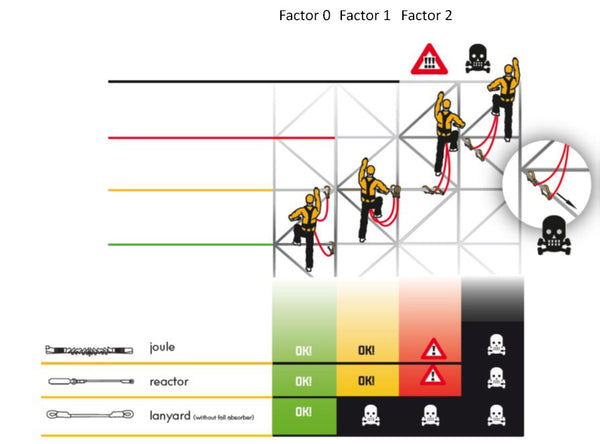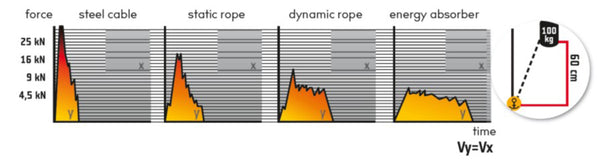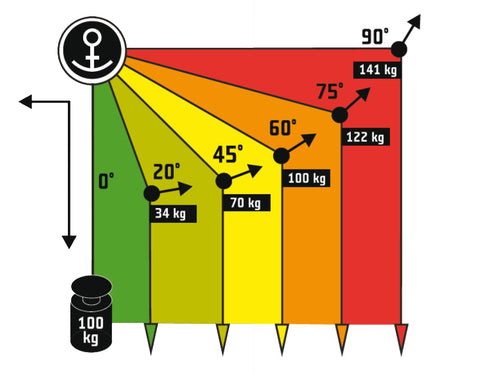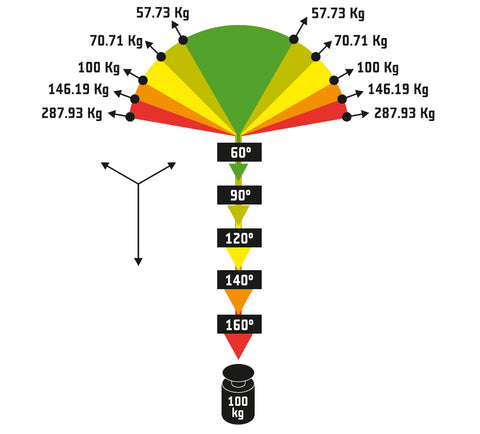Rope access workers and technicians must understand the risk of falling when working at heights. There are some basic concepts behind the risk of falling and we will discuss some of them in this article. We will quickly look at the basic knowledge behind the fall factor, its formula, and its values.

Then we will move on to the impact force and how it affects the safety of workers. At the end we will discuss load bearing of different anchoring systems and why you should always think about degrees when anchoring.

Let’s dive in.

## Fall factor – basic knowledge

First, let’s explain what the fall factor is.

Fall factor is the ratio of the height worker falls and the rope length available to absorb the energy of the fall.

### Fall factor formula

We can calculate the fall factor using following formula:

f=h/L, where

• f is the fall factor (its theoretical value),
• h is the height a worker/climber falls and
• L is the rope length (available to absorb the energy of the fall)

From the formula you can derive that the severity of the fall does not depend only on the fall height, but also on the rope length – the longer the rope, the more energy of the fall it can absorb.

### Fall factor values

The fall factor values can be between 0 and 2. Zero is the lowest possible fall factor and 2 is the highest. The higher the fall factor, the higher the severity of the fall.

For rope access technicians and for workers at heights the fall factor should not exceed a value of 1. If the fall factor is higher than 1, workers should implement strategies to minimize the fall factor through equipment, such as back up devices, etc.

## Fall factor when working at height

Now, let’s look in more detail on fall factor for workers at height and rope access technicians. The distance workers at heights can fall is largely determined by the position of the anchor point. A higher anchor point reduces the fall distance and reduce the risk of possible injury in case of the fall. When workers move above this anchor, the severity of the potential fall increases.

If the anchor point is above the worker and the line is stretched, the fall distance is shorter, and the fall factor is around 0. If the anchor point is at the height of worker’s shoulders, the fall distance can be at least the same as the full length of the rope, so in this case, the value of fall factor is around 1. However, the most dangerous situation is when the anchor point is placed at the height of the worker’s feet (or below). In this case, the fall distance is at least twice the length of the rope and the fall factor has dangerously high value of 2.

All these situations are illustrated in the picture below from Singing Rock.When safeguarding an ascent using a fall arrest type "Y" (fall arrest type Y consists of two lanyards branching off from an energy absorber to form "Y" shape), it is necessary to move to the level of fall factor 1 or lower. It means that the connectors connected to the fall absorber during the ascent do not "fall" below the connection point of the harness, in which the absorber is connected to the harness.

Only connectors in conformance with European Standard EN 362 should be used as joining devices in fall arrest systems. Similarly, the fall absorber should comply with EN 355 and the harness with EN 361.

## Impact force

The impact force determines the maximum value of force generated during fall arrest of the object by the safety chain. The value of the impact force has a direct impact on the safety of at height workers. Ideally, we avoid a large impact force if we reduce it to the lowest level possible. A Fall arrest system – a system that is designed to arrest a fall while limiting the impact force – must ensure that the impact force does not exceed 6 kN.

The impact force and its distribution over time depend on the type of the safety chain which is used for energy absorption, as you can see in the picture below.Steel cables have almost no capacity for energy absorption and cannot be used to arrest a fall. A static rope and dynamic rope have low capacity for energy absorption, and the impact force is in both cases above the recommended value of 6 kN. That is why a fall arrest system usually has an energy absorber. A fall arrest system without the fall absorber cannot absorb enough energy to keep the impact force below recommended values and expose its users to the risk of injury.

## Anchoring

As we mentioned, the value of the fall factor depends on the position of the anchor point when working at height.  A higher anchor point means a lower fall factor, as it reduces the fall distance. However, there is one other thing that must be considered: the load of the anchoring point.

This is especially important because the force on the anchoring point can be much higher than the weight of the worker. These situations are hazardous and should be avoided. We will briefly look at two cases when this could happen.

### Load of continuous anchoring point

Load of continuous anchoring point depends on the angle. When working at height, rope deviation techniques around hazards and obstacles are often common but should be kept to lower than 60 degrees.As you can see in the picture above, if the angle is greater than 60 degrees, the load on the anchor is greater than the load on the rope. Deviation angles of higher than 60 degrees are considered extremely dangerous when working at height as this overloads the anchor point and reduces the safe level of impact force.

A load-sharing anchor consists of two or more individual anchors that are joined together at a main anchor point. Together they form an anchoring system. The main difference of this from a single anchor point is that the load is distributed between the anchors. If any single anchor point fails, the other anchor point will take the load of the force.

When using a load-sharing anchor, it is necessary to take into account the angle of supporting elements – otherwise known as the V-angle.

As you can see in the picture below, the greater the V-angle, the greater the load of each individual anchor.If the V-angle is greater than 120 degrees (orange and red in the illustration above), the load on each anchor is greater than the load on the rope – which is a very dangerous situation that should be avoided.

## Conclusion

In conclusion, always go for a fall factor of 1 or lower when working at height. Also, never leave a connector below you and always think about the degrees when anchoring - 60 or less degrees when using continuous anchor point, and 120 or less degrees when using load-sharing anchor.